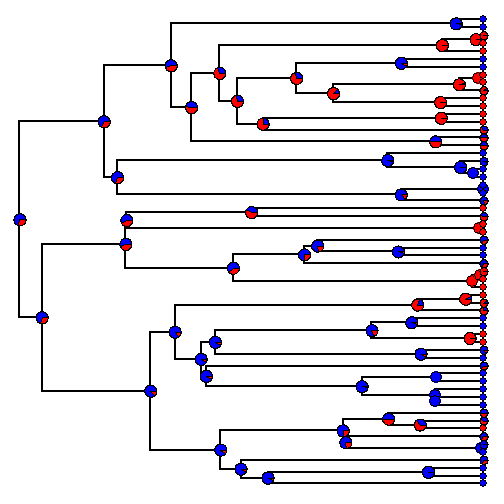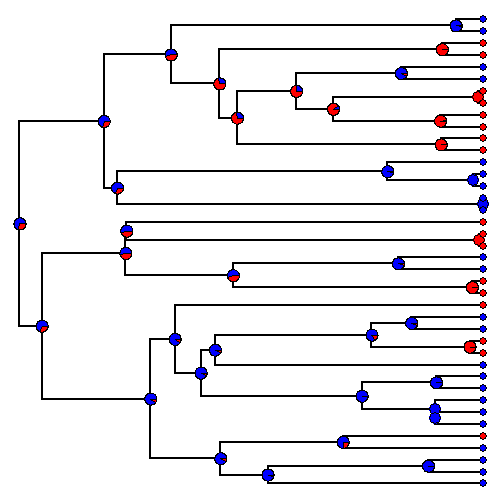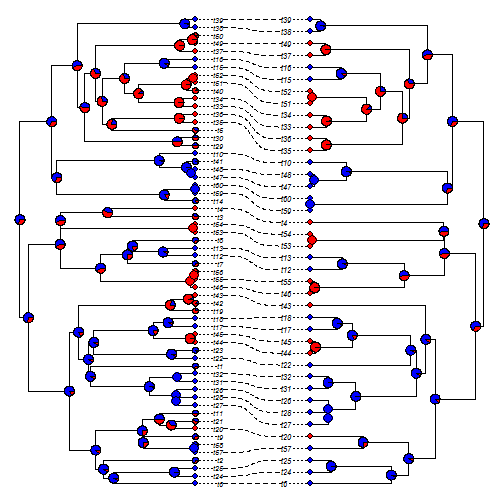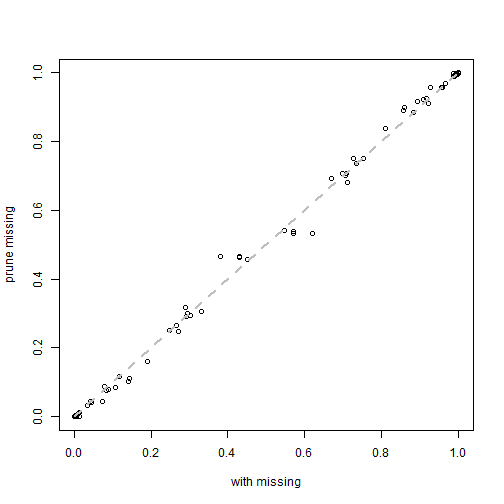## Sunday, October 23, 2016

### Stochastic mapping discrete character histories with missing data at some tips

Stochastic mapping in phytools permits some tips to have unknown states. This is done by using a prior probability distribution on the tips that is flat across all possible states. This leaves the posterior probabilities at internal nodes largely unaffected compared to just dropping said tips from the tree. (Although I do not know for sure that they could not theoretically be affected, it is hard to see how.)

Here's an example using simulated data.

``````library(phytools)
library(phangorn)
``````

Simulate data including missing tip values for some taxa:

``````## simulate tree & data
N<-60 ## number of tips
n.miss<-20 ## number with missing values
tree<-pbtree(n=N,scale=1)
Q<-matrix(c(-1,1,1,-1),2,2)
rownames(Q)<-colnames(Q)<-c("a","b")
x<-sim.history(tree,Q)\$states
``````
``````## Done simulation(s).
``````
``````X<-to.matrix(x,c("a","b"))
## missing
missing<-sample(tree\$tip.label,n.miss)
tips<-sapply(missing,function(x,y) which(y==x),
y=tree\$tip.label)
X[missing,]<-rep(0.5,2)
``````

Now perform stochastic mapping:

``````## stochastic mapping
## with missing
m1<-make.simmap(tree,X,nsim=500)
``````
``````## make.simmap is sampling character histories conditioned on the transition matrix
##
## Q =
##            a          b
## a -0.8958661  0.8958661
## b  0.8958661 -0.8958661
## (estimated using likelihood);
## and (mean) root node prior probabilities
## pi =
##   a   b
## 0.5 0.5
``````
``````## Done.
``````
``````plot(sum1<-summary(m1),colors=setNames(c("blue","red"),c("a","b")),
ftype="off")
````````````## without missing
m2<-make.simmap(drop.tip(tree,missing),
X[setdiff(tree\$tip.label,missing),],
nsim=500)
``````
``````## make.simmap is sampling character histories conditioned on the transition matrix
##
## Q =
##           a         b
## a -0.895866  0.895866
## b  0.895866 -0.895866
## (estimated using likelihood);
## and (mean) root node prior probabilities
## pi =
##   a   b
## 0.5 0.5
``````
``````## Done.
``````
``````plot(sum2<-summary(m2),colors=setNames(c("blue","red"),c("a","b")),
ftype="off")
``````We can also visualize the concordance of internal node reconstructions using `cophylo`:

``````obj<-cophylo(tree,drop.tip(tree,missing),rotate=FALSE)
tiplabels.cophylo(pie=sum1\$tips,
piecol=setNames(c("blue","red"),c("a","b")),
which="left",cex=0.3)
nodelabels.cophylo(pie=sum1\$ace,
piecol=setNames(c("blue","red"),c("a","b")),
which="left",cex=0.5)
tiplabels.cophylo(pie=sum2\$tips,
piecol=setNames(c("blue","red"),c("a","b")),
which="right",cex=0.3)
nodelabels.cophylo(pie=sum2\$ace,
piecol=setNames(c("blue","red"),c("a","b")),
which="right",cex=0.5)
``````Finally, we can compare the nodes by mapping one to the other across the two analyses, and then comparing the posterior probabilities directly:

``````## compare nodes
M<-matchNodes(tree,drop.tip(tree,missing),"distances")
plot(sum1\$ace[as.character(M[!is.na(M[,2]),1]),],
sum2\$ace,xlab="with missing",ylab="prune missing")
lines(c(0,1),c(0,1),lty="dashed",col="grey",lwd=2)
lines(c(0,1),c(0,1),lty="dashed",col="grey",lwd=2)
``````Note that for the case of computing the likelihood of a tree given a data pattern at the tip, we would set the probabilities of each state equal to 1.0. Here I'm computing the likelihood of a particular character pattern at the nodes & missing tips (& a model), given the data for the tips so I have set the prior probabilities for the missing nodes to be 1/(number of states). I'm not sure this makes a difference (other than numerically affecting the computed likelihood); however I suppose it could.

#### 1 comment:

1.This comment has been removed by the author.

Note: due to the very large amount of spam, all comments are now automatically submitted for moderation.